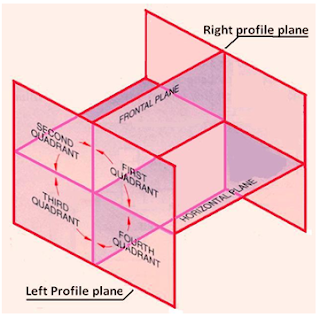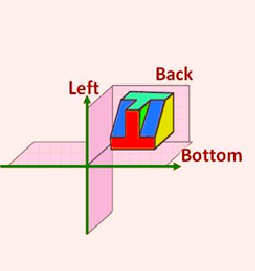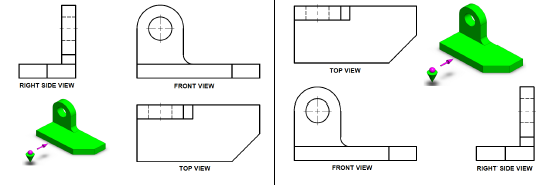# First Angle vs Third Angle

Collection of 2D drawings which enables you to have a complete representation of an object is called orthographic projection. Collection of 2D drawings consist of six orthographic views (Top, Bottom, Right, Left, Front and Back view) also called as six principle views.

From these six orthographic views front, right side and top view are most commonly used to represent the orthographic projection of an object. For getting the orthographic views from a 3D object there are two main types of projections used one is First angle projection and other is Third angle projection.

Main objective of both of these projections angle is to provide detail 2D drawings of 3D object but there are some differences in the way one can get the projects by using one on these methods. Following is a comparison of first angle vs third angle and a comprehensive differences between First angle and third angle projection.

## Quadrant (first angle vs third angle)

To start getting projection first divides the plane into four quadrants. For the first angle projection object is paced in the first quadrant and for the third angle projection object is placed is third quadrant.Quadrants of First Angle vs Third Angle ProjectionQuadrants of First Angle ProjectionQuadrants of Third Angle Projection

## Object Placement (difference between first and third angle projection)

To get first angle projection object is place in between the plane of projection and observer and for the third angle projection plane of projection is placed between the object and observer.Object Placement of First Angle and Third Angle Projection

## State of Projection Plane (first and third angle projection)

In first angle of projection plane of projection is taken solid while in third angle of projection it is taken as transparent.

## Views Sequence (First Angle vs Third Angle)

In first angle projection right view come in left of front view and top view come at the bottom of front view while in third angle projection right view come on right side of front view and top view comes at the top of front view.View Sequence of First Angle vs Third Angle Projection

## Symbols First Angle vs Third AngleSymbol of First Angle vs Third Angle ProjectionFirst Angle vs Third Angle

1.First Angle difination

2.3.4.THANK YOU SO MUCH...

5.this is one of the best ways to remember, first angle projection - planes are assumed opaque, third angle projection - planes are assume transparent